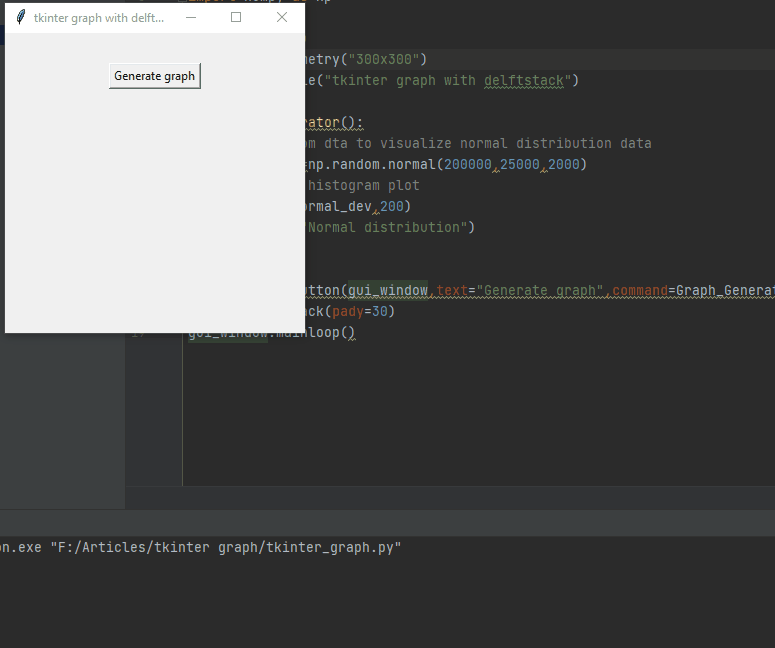# Tkinter 图

Matplotlib 是 Python 的绘图库，它允许我们从数据中创建一些漂亮的图形；这用于数据科学领域和我们需要可视化数据分析的应用程序。使用 Matplotlib，我们可以在 Python 中进行一些漂亮的可视化。

## 在 Python 中使用 Tkinter 创建 Matplotlib 图形

``````pip install numpy
pip install matplotlib
``````

``````from tkinter import *
import matplotlib.pyplot as plt
import numpy as np
``````

``````def Graph_Generator():
# get random dta to visualize normal distribution data
normal_dev=np.random.normal(200000,25000,2000)
# Create a histogram plot
plt.hist(normal_dev,200)
plt.title("Normal distribution")
plt.show()
``````

``````graph_button=Button(gui_window,text="Generate graph",command=Graph_Generator)
``````

``````from tkinter import *
import matplotlib.pyplot as plt
import numpy as np
gui_window=Tk()
gui_window.geometry("300x300")
gui_window.title("tkinter graph with delftstack")
def Graph_Generator():
# get random dta to visualize normal distribution data
normal_dev=np.random.normal(200000,25000,2000)
# Create a histogram plot
plt.hist(normal_dev,200)
plt.title("Normal distribution")
plt.show()
graph_button=Button(gui_window,text="Generate graph",command=Graph_Generator)